#Function Repository Resource:

# TupleIndex

Compute the index of a given tuple of non-negative integers

Contributed by: Ed Pegg Jr
 ResourceFunction["TupleIndex"][tuple] returns the index of tuple as it would appear in a list of all integer tuples of the same length, sorted by the maximal element.

## Details and Options

A tuple with largest element n will be ordered after tuples that have all elements less than n.
In case of ties, tuples with largest element n have Sort ordering.

## Examples

### Basic Examples (6)

Get the index of a 3-tuple:

 In:=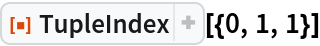Out=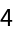Get the index of a 3-tuple with a larger element:

 In:=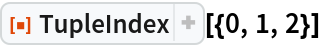Out=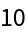Show by example how element sizes and positions affect ordering:

 In:=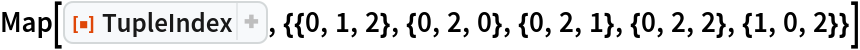Out=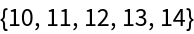Indices of 2-tuples sorted by maximal element:

 In:=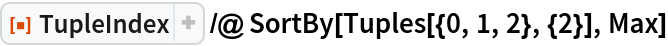Out=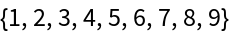Indices of 3-tuples sorted by maximal element:

 In:=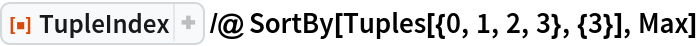Out=Show the tuples as they would be sorted by TupleIndex:

 In:=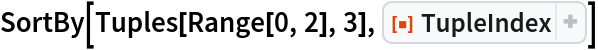Out=Find indices of some larger k-tuples:

 In:=Out=### Properties and Relations (2)

Use Tuples to produce 3-tuples sorted by maximal element:

 In:=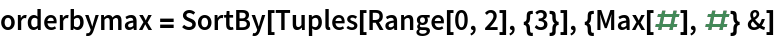Out=TupleIndex is based on this ordering:

 In:=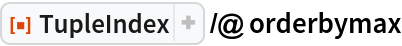Out=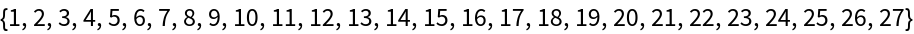### Neat Examples (2)

Find the index of a large 6-tuple:

 In:=Out=Find the index of a large 111-tuple:

 In:=Out=